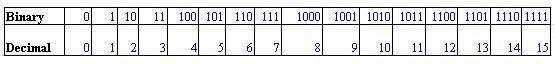# Binary Formula

The Binary Numbering System is the most fundamental numbering system in mathematics as well as in all digital and computer-based systems. Binary numbers follow the same set of rules as the decimal numbering system.

The Binary Numbering System contains only 0’s and 1’s. A binary number such as 101100101 is expressed with a string of 0’s and 1’s with each digit having a value twice that of the previous digit.

While, writing the binary numbers you need to signify the number is binary (base 2), for instance: let’s take the value 101. As it is written, it would be hard to know to work out whether it is a binary or decimal value.

The weight of each binary bit relies on its relative position within the number. The binary formula to convert decimal to binary is given below:

Binary to Decimal Formula

$$\begin{array}{l}Decimal Number = n^{th} bit \times 2^{n-1}\end{array}$$

To convert binary to decimal the following chart is used and binary is noted as per the given decimal number.Converting binary to a decimal number or decimal to binary number is an easy task. But, you need to be careful not to mix up the two sets of numbers. For instance, if you write the digits 10 on the page it could mean the number “ten” if we assume it to be a decimal number, or it could equally be a “1” and a “0” together in binary, which is equal to the number two in the weighted decimal format from above table.

So, for instance, if you use a binary number string it should add the subscript “2” to denote a base 2 number so the binary number would be written as 102.

Likewise, if it was a standard decimal number it would add the subscript “10” to denote a base 10 and written as 1010.

0 + 0 = 0

0 + 1 = 1

1 + 0 = 1

1 + 1 = 0(CARRY 1)

1 + 1 + 1 = 11(CARRY 1)

Binary Numbers Subtraction

0 – 0 = 0

0 – 1 = 1 (with a borrow of 1 )

1 – 0 = 1

1 – 1 = 0

Binary Examples

Here are some examples of adding and subtracting binary numbers.

Example 1: Add the following binary numbers:

111002 and 101102.

Solution:

Given binary numbers are: 11100 and 10110

11100 +

10110

———–

110010

———–

Example 2: Subtract (10001)2 from (11101)2

Solution:

Given digits are 11101 and 10001

11101

-10001

———-

01100

———-

 More topics in Binary Formula Binary to Decimal Formula Decimal to Binary Formula